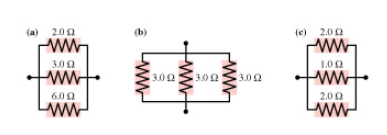# Problem: A) What is the equivalent resistance of group (a) of resistors shown in the figure? B)  What is the equivalent resistance of group (b) of resistors shown in the figure?Express your answer using two significant figures. Please show work.

###### FREE Expert Solution

For resistors in parallel:

$\overline{)\frac{\mathbf{1}}{{\mathbf{R}}_{\mathbf{e}\mathbf{q}}}{\mathbf{=}}\frac{\mathbf{1}}{{\mathbf{R}}_{\mathbf{1}}}{\mathbf{+}}\frac{\mathbf{1}}{{\mathbf{R}}_{\mathbf{2}}}{\mathbf{+}}{\mathbf{.}}{\mathbf{.}}{\mathbf{.}}{\mathbf{+}}\frac{\mathbf{1}}{{\mathbf{R}}_{\mathbf{n}}}}$

90% (300 ratings)###### Problem DetailsA) What is the equivalent resistance of group (a) of resistors shown in the figure?

B)  What is the equivalent resistance of group (b) of resistors shown in the figure?

Express your answer using two significant figures. Please show work.

Frequently Asked Questions

What scientific concept do you need to know in order to solve this problem?

Our tutors have indicated that to solve this problem you will need to apply the Solving Resistor Circuits concept. You can view video lessons to learn Solving Resistor Circuits. Or if you need more Solving Resistor Circuits practice, you can also practice Solving Resistor Circuits practice problems.

What professor is this problem relevant for?

Based on our data, we think this problem is relevant for Professor Zhou's class at UT.